# Class 9 Maths Worksheet for Quadrilaterals

Given below are the Class 9 Maths Worksheet for Quadrilaterals
a. Proof questions
b. Difficult problems
Question 1
ABCD is a parallelogram and AP and CQ are perpendiculars from vertices A and C on diagonal BD. Show that :
(i) $\Delta APB \cong \Delta CQD$
(ii) AP = CQ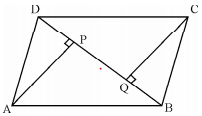In Triangle APB and CQD
AD=BC ( opposite sides of the Parallelogram)
$\angle ADP=\angle QBC$ ( Alternate angles between parallel lines)
$\angle APB= \angle CQD =90$
Hence by AAS Congruency
$\Delta APB \cong \Delta CQD$
Now from CPCT of Congruent triangles
AP = CQ

Question 2
The angles of a quadrilateral are in the ratio 3 : 5 : 7 : 9. Find the angles of the quadrilateral.

Angles are 3x,5x,7x,9x
So
$3x+5x+7x+9x=360$
$24x=360$
x=15

So angles are
45, 75,105, 145

Question 3
In a quadrilateral ABCD, AO and BO are the bisectors of $\angle A \; and \; \angle B$ respectively. Prove that $\angle AOB = \frac {1}{ 2} (\angle C + \angle D)$

Now we know that
$\angle A + \angle B + \angle C + \angle D=360$
$\angle C + \angle D=360 - (\angle A + \angle B)$
$\frac {1}{ 2} (\angle C + \angle D)=180 - \frac {1}{2}(\angle A + \angle B)$ -(1)
In Triangle AOB
$\angle AOB + \frac {1}{2} \angle A + \frac {1}{2} \angle B=180$
$\angle AOB= 180 - \frac {1}{2}(\angle A + \angle B)$ -(2)
From (1) and (2)
$\angle AOB = \frac {1}{ 2} (\angle C + \angle D)$

Question 4
In the figure, ABCD is a parallelogram. E is the mid-point of BC. DE and AB when produced meet at F. Prove that AF = 2AB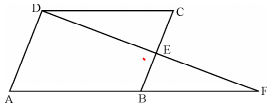Question 5
ABCD is a square and on the side DC, an equilateral triangle is constructed. Prove that
(i) AE = BE
(ii) $\angle DAE = 15^0$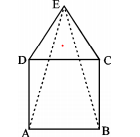(i) In triangle ADE and BCE ,
$\angle ADC = \angle BCD = 90$
$\angle EDC = \angle ECD$ as triangle ECD is an equilateral triangle.
Therefore
$\angle EDA = \ angle ECB$
AD = BC ( same sides of a square )
DE = CE ( equal sides of an equilateral triangle)
By SAS both are congruent
By CPCT
AE = BE
$\angle DAE= \angle DEA$ as both the sides are equal
Also
$\angle EDA= 90 + 60=150$
Now
$\angle DAE + \angle DEA + \angle EDA=180$
Hence $\angle DAE = 15^0$

Question 6
ABCD is a rectangle in which diagonal BD bisects ∠B. Show that ABCD is a square.
Question 7
If ABCD is a trapezium in which AB || CD and AD = BC, prove that ∠A = ∠B
Question 8
In the figure, diagonal BD of parallelogram ABCD bisects ∠B. Show that it bisects ∠D also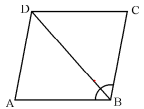Question 9
E and F are points on diagonal AC of a parallelogram ABCD such that AE = CF. Show that BFDE is a parallelogram.
Question 10
The two opposite angles of a parallelogram are $(3y - 10)^0$ and $(2y + 35)^0$. Find the measure of all the four angles of the parallelogram

Opposite angles in a parallelogram are equal
$(3y - 10)=(2y + 35)$
$y=45$
So opposite angles are 125° and 125°
Other will be 65° and 65°

Question 11
ABCD is a rhombus in which AC = 16 cm and BC = 10 cm. Find the length of the diagonal BD.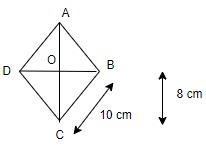$OB^2 + OC^2=BC^2$
$OB^2=BC^2-OC^2= 100-64$
$OB=6$
Therefore BD=12 cm

Question 12
Diagonals of a quadrilateral ABCD bisect each other. If ∠A = 35°, then ∠B = 145°. Is it true ? Justify your answer.
Question 13
Can $\angle 95^0 \; ,\; 70^0 \; ,\; 110^0 \; and \; 80^0$ be the angles of a quadrilateral ? Why or why not ?

Sum of the angles of the quadrilateral=360
Here It is not, so this is not possible

Question 14
Prove that quadrilateral formed by bisectors of the angles of a parallelogram is a rectangle.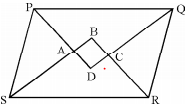Question 15
Show that the line segments joining the mid-points of opposite sides of quadrilateral bisect each other.
Question 16
ABCD is a parallelogram in which X and Y are the mid-points of AB and CD. AY and DX are joined which intersect each other at P. BY and CX are also joined which intersect each other at Q. Show that PXQY is a parallelogram
Question 17
Show that if the diagonals of a quadrilateral bisect each other at right angles, then it is a rhombus
Question 18
If one angle of a parallelogram is a right angle, it is a rectangle. Prove.
Question 19.
In a parallelogram ABCD, $\angle D = 135^0$, determine the angles measures of $\angle A \; and \; \angle B$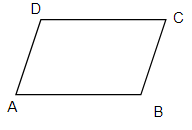Opposite angles in a parallelogram are equal.
Therefore
∠ B=∠ D=135°
Adjacent angles in a parallelogram are supplementary
∠ A+ ∠ B=180°
∠A=180-135
∠A=45 °

Question 20.
ABCD is a parallelogram in which $\angle A = 70^0$. Compute $\angle B \; ,\; \angle C \;and \; \angle D$Opposite angles in a parallelogram are equal.
Therefore
∠ A=∠ C=70°
Adjacent angles in a parallelogram are supplementary
∠ A+ ∠ B=180°
∠B=180-70
∠B=110 °
Again
∠ B=∠ D=110°

Question 21.
ABCD is a parallelogram in which $\angle DAB = 75^0$ and $\angle DBC = 60^0$. Compute $\angle CDB \; and \; \angle ADB$.

The situation is depicted in below figure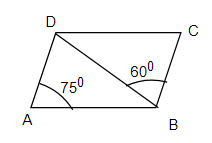Opposite angles in a parallelogram are equal.
Therefore
∠ BCD = ∠ DAB=75°
In Δ BDC
∠ BCD + ∠ DBC + ∠ CDB =180°
∠ CDB= 180 -75-60=45°
Now alternat angles are equal

Question 22.
ABCD is a parallelogram and X, Y are the mid- points of sides AB and DC respectively. Show that in parallelogram ABCD, AXCY is a parallelogram.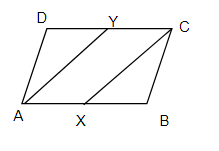AB=CD ( Opposite side of parallelogram)
Since X and Y are mid-points of AB and DC respectively.
AX = 1/2 AB and CY = 1/2 DC
Therefore
AX = CY and AX || CY.
One pair of opposite sides is parallel and equal
Hence AECF is a parallelogram.

Question 23.
The sides AB and CD of a parallelogram ABCD are bisected at E and F. Prove that EBFD is a parallelogram.
Question 24.
ABCD is a square E, F, G and H are points on AB, BC, CD and DA respectively. Such that AE = BF = CG = DH. Prove that EFGH is a square.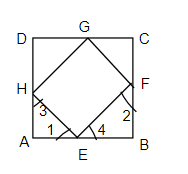Given: AE = BF = CG = DH
Since all the sides are equal in Square
Therefore
BE=CF=DG=AH
Now in Δ AHE and BEF
AE=BF
AH=BE
∠ A = ∠ B
By SAS congruence
Δ AEH ≅ Δ BFE
Therefore by CPCT
HE=EF
∠ 1 = ∠ 2
∠ 3 = ∠ 4
Now but ∠ 1 + ∠ 3=90
As ∠ 3 = ∠ 4
∠ 1 + ∠ 4=90°
i.e ∠ E= 90°
Similarly we can proof for other sides and angles
Hence EFGH is a square

Question 25.
ABCD is a rhombus, EABF is a straight line such that EA = AB = BF. Prove that ED and FC when produced meet at right angles.

## Summary

This Quadrilaterals Worksheet for Class 9 Maths with answers is prepared keeping in mind the latest syllabus of CBSE . This has been designed in a way to improve the academic performance of the students. If you find mistakes , please do provide the feedback on the mail.